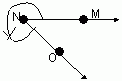Name: ___________________Date:___________________

 Email us to get an instant 20% discount on highly effective K-12 Math & English kwizNET Programs!

### MEAP Preparation - Grade 5 Mathematics3.19 Classifying Angles

 Acute Angle: An angle whose measure is less than 90 degrees and greater then zero degrees is called an acute angle. 00 < mĐA < 900 Right Angle: An angle whose measure is 90 degrees is called right angle. mĐA = 900 Obtuse Angle: An angle whose measure is greater than 90 degrees and less than 180 degrees is called obtuse angle. 900 < mĐA < 1800 Straight Angle: An angle whose measure is 180 degrees called a straight angle. mĐA = 1800 Reflex Angle: An angle whose measure is greater than 180 degrees and less than 360 degrees is called a reflex angle. 1800 < mĐA < 3600 Complete Angle: An angle whose measure is 360 degrees is called a complete angle. mĐA = 3600 Zero Angle: If the measure of an angle is zero it is called a zero angle. mĐA = 00 Directions: Answer the following questions. Also draw kinds of angles, measure them using a protractor and label them.
 Q 1: What is the type of the angle shown?Right AngleAcute AngleObtuse Angle Q 2: What is the type of the angle shown?Right AngleObtuse AngleAcute Angle Q 3: What is the type of the angle shown?Complete AngleRight AngleAcute Angle Q 4: What is the type of the angle shown?Straight AngleComplete AngleZero Angle Q 5: What is the type of the angle shown?Reflex AngleAcute AngleRight angle Q 6: What is the type of the angle shown?Right AngleComplete AngleAcute angle Question 7: This question is available to subscribers only! Question 8: This question is available to subscribers only!